• Get access

Do you like the article?
Share it with others -

Use new possibilities of MetaTrader 5

# Machine Learning: How Support Vector Machines can be used in Trading

17 December 2012, 10:45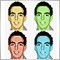6
19 096

### What is a Support Vector Machine?

A support vector machine is a method of machine learning that attempts to take input data and classify into one of two categories. In order for a support vector machine to be effective, it is necessary to first use a set of training input and output data to build the support vector machine model that can be used for classifying new data.

A support vector machine develops this model by taking the training inputs, mapping them into multidimensional space, then using regression to find a hyperplane (a hyperplane is a surface in n-dimensional space that it separates the space into two half spaces) that best separates the two classes of inputs. Once the support vector machine has been trained, it is able to assess new inputs with respect to the separating hyperplane and classify it into one of the two categories.

A support vector machine is essentially an input/output machine. A user is able to put in an input, and based on the model developed through training, it will return an output. The number of inputs for any given support vector machine theoretically ranges from one to infinity, however in practical terms computing power does limit how many inputs can be used. If for example, N inputs are used for a particular support vector machine (the integer value of N can range from one to infinity), the support vector machine must map each set of inputs into N-dimensional space and find a (N-1)-dimensional hyperplane that best separates the training data.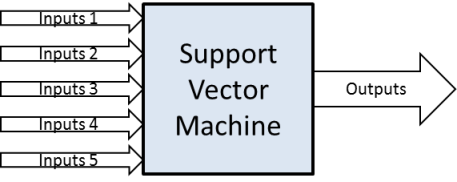Figure 1. Support Vector Machines are input/output machines

The best way to conceptualize how a support vector machine works is by considering the two dimensional case. Assume we want to create a support vector machine that has two inputs and returns a single output that classifies the data point as belonging to one of two categories. We can visualize this by plotting it on a 2-dimensional chart such as the chart below.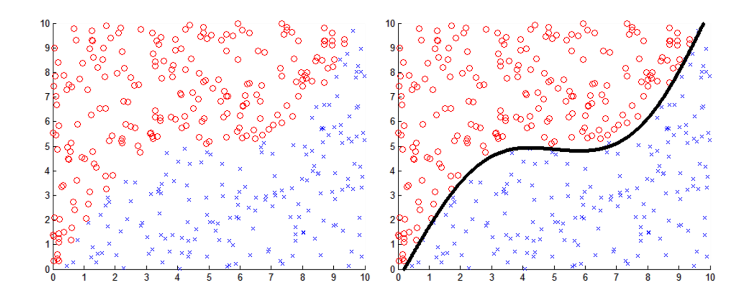Figure 2. Left: Support vector machine inputs mapped to a 2D chart. The red circles and blue crosses are used to denote the two classes of inputs.

Figure 3. Right: Support vector machine inputs mapped to a 2D chart. The red circles and blue crosses are used to denote the two classes of inputs with a black line indicating the separating hyperplane.

In this example, the blue crosses indicate data points that belong to category 1 and the red circles that represent data points that belong to category 2. Each of the individual data points has unique input 1 value (represented by their position on the x-axis) and a unique input 2 value (represented by their position on the y-axis) and all of these points have been mapped to the 2-dimensional space.

A support vector machine is able to classify data by creating a model of these points in 2 dimensional space. The support vector machine observes the data in 2 dimensional space, and uses a regression algorithm to find a 1 dimensional hyperplane (aka line) that most accurately separate the data into its two categories. This separating line is then used by the support vector machine to classify new data points into either category 1 or category 2.

The animation below illustrates the process of training a new support vector machine. The algorithm will start by making a random guess finding a separating hyperplane, then iteratively improve the accuracy of the hyperplane. As you can see the algorithm starts quite aggressively, but then slows down as it starts to approach the desires solution.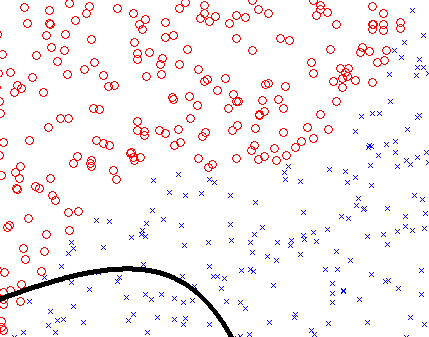Figure 4. An animation showing a support vector machine training. The hyperplane progressively converges on the ideal geometry to separate the two classes of data

Higher Dimensions

The 2-dimensional scenario above presented allows us to visualize the the process of a support vector machine, however it is only able to classify a data point using two inputs. What if we want to use more inputs? Thankfully, the support vector machine algorithm allows us to do the same in higher dimensions, though it does become much harder to conceptualize.

Consider this, you wish to create support vector machine that takes 20 inputs and can classify any data point using these inputs into either category 1 or category 2. In order to do this, the support vector machine needs to model the data in 20 dimensional space and use a regression algorithm to find a 19 dimensional hyperplane that separates the data points into two categories. This gets exceedingly difficult to visualize as it is hard for us to comprehend anything above 3-dimensions, however all that you need to know is that is works in exactly the same way as it does for the 2-dimensional case.

### How do Support Vector Machines Work? Example: Is It A Schnick?

Imagine this hypothetical scenario, you are a researcher investigating a rare animal only found in the depths of the Arctic called Shnicks. Given the remoteness of these animals, only a small handful have ever been found (let's say around 5000). As a researcher, you are stuck with the question... how can I identify a Schnick?

All you have at your disposal are the research papers previously published by the handful of researchers that have seen one. In these research papers, the authors describe certain characteristics about the Schnicks they found, i.e. height, weight, number of legs, etc. But all of these characteristics vary between the research papers with no discernible pattern...

How can we use this data to identify a new animal as a schnick?

One possible solution to our problem is to use a support vector machine to identify the patterns in the data and create a framework that can be used to classify animals as either a schnick or not a schnick. The first step is to create a set of data that can be used to train your support vector machine to identify schnicks. The training data is a set of inputs and matching outputs for the support vector machine to analyze and extract a pattern from.

Therefore, we must decide what inputs will be used and how many. Theoretically, we can have as many inputs as we want, however this can often lead to slow training (the more inputs you have the more time it takes the support vector machine to extract patterns). Also, you want to choose inputs values that will tend to be relatively consistent across all schnicks. For example, height or weight of the animal would be a good example of an input because you would expect that this would be relatively consistent across all schnicks. However, the average age of an animal would be a poor choice of input because you would expect the age of animals identified would all vary considerably.

For this reason, the following inputs were chosen:

• Height
• Weight
• The number of legs
• The number of eyes
• The length of the animal's arms
• The animals average speed
• The frequency of the animals mating call

With the inputs chosen, we can start to compile our training data. Effective training data for a support vector machine must meet certain requirements:

• The data must have examples of animals that are schnicks
• The data must have examples of animals that are not schnicks

In this case we have the research papers of scientist that have successfully identified a schnick and listed their properties. Therefore we can read these research papers and extract the data under each of the inputs and allocate an output of either true or false to each of the examples. The training data in this case may look similar to the table below.

Training Samples height [mm] weight [kg] N_legs N_eyes L_arm [mm] av_speed [m/s] f_call [Hz] Schnick (true/false)
Example 1 1030 45 8 3 420 2.1 14000 TRUE
Example 2 1010 42 8 3 450 2.2 14000 TRUE
Example 3 900 40 7 6 600 6 13000 FALSE
Example 4 1050 43 9 4 400 2.4 12000 TRUE
Example 5 700 35 2 8 320 21 13500 FALSE
Example 6 1070 42 8 3 430 2.4 12000 TRUE
Example 7 1100 40 8 3 430 2.1 11000 TRUE
Example N ... ... ... ... ... ... ... ...

Table 1. Example table of schnick observations

Once we have gathered the data for all of our training inputs and outputs, we can use it to train our support vector machine. During the training process, the support vector machine will create a model in seven dimensional space that can be used to sort each of the training examples into either true or false. The support vector machine will continue to do this until it has a model that accurately represents the training data (within the specified error tolerance). Once training is complete, this model can be used to classify new data points as either true or false.

### Does the Support Vector Machine Actually Work?

Using the Schnick scenario, I have written a script that tests how well a support vector machine can actually identify new schnicks. To do this, I have used the “Support Vector Machine Learning Tool” function Library that can be downloaded from the Market.

To model this scenario effectively, we need to first decide what are the actual properties of a Schnick. The properties I have assumed in this case have been listed in the table below. If an animal satisfies all of the criteria below, then it is a Schnick...

Parameter Lower Range Upper Range
height [mm] 1000 1100
weight [kg] 40 50
N_legs 8 10
N_eyes 3 4
L_arm [mm] 400 450
av_speed [m/s] 2 2.5
f_call [Hz] 11000 15000

Table 2. Summary of parameters that define a schnick

Now that we have defined our Schnick, we can use this definition to experiment with support vector machines. The first step is to create a function that is able to take the seven inputs for any given animal and return the actual classification of the animal as a schnick or not. This function will be used to generate training data for the support vector machine as well as assess the performance of it at the end. This can be done using the function below;

```//+------------------------------------------------------------------+
//| This function takes the observation properties of the observed
//| animal and based on the criteria we have chosen, returns true/false whether it is a schnick
//+------------------------------------------------------------------+
bool isItASchnick(double height,double weight,double N_legs,double N_eyes,double L_arm,double av_speed,double f_call)
{
if(height   < 1000  || height   > 1100)  return(false);   // If the height is outside the parameters > return(false)
if(weight   < 40    || weight   > 50)    return(false);   // If the weight is outside the parameters > return(false)
if(N_legs   < 8     || N_legs   > 10)    return(false);   // If the N_Legs is outside the parameters > return(false)
if(N_eyes   < 3     || N_eyes   > 4)     return(false);   // If the N_eyes is outside the parameters > return(false)
if(L_arm    < 400   || L_arm    > 450)   return(false);   // If the L_arm  is outside the parameters > return(false)
if(av_speed < 2     || av_speed > 2.5)   return(false);   // If the av_speed is outside the parameters > return(false)
if(f_call   < 11000 || f_call   > 15000) return(false);   // If the f_call is outside the parameters > return(false)
return(true);                                             // Otherwise > return(true)
}```

The next step in the process is to create a function that can generate the training inputs and outputs. Inputs in this case will be generated by creating random numbers within a set range for each of the seven input values. Then for each of the sets of random inputs generated, the isItASchnick() function above will be used to generate the corresponding desired output. This is done in the function below:

```//+------------------------------------------------------------------+
//| This function takes an empty double array and an empty boolean array,
//| and generates the inputs/outputs to be used for training the SVM
//+------------------------------------------------------------------+
void genTrainingData(double &inputs[],bool &outputs[],int N)
{
double in[];                    // Creates an empty double array to be used for temporarily storing the inputs generated
ArrayResize(in,N_Inputs);       // Resize the in[] array to N_Inputs
ArrayResize(inputs,N*N_Inputs); // Resize the inputs[] array to have a size of N*N_Inputs
ArrayResize(outputs,N);         // Resize the outputs[] array to have a size of N
for(int i=0;i<N;i++)
{
in=    randBetween(980,1120);      // Random input generated for height
in=    randBetween(38,52);         // Random input generated for weight
in=    randBetween(7,11);          // Random input generated for N_legs
in=    randBetween(3,4.2);         // Random input generated for N_eyes
in=    randBetween(380,450);       // Random input generated for L_arms
in=    randBetween(2,2.6);         // Random input generated for av_speed
in=    randBetween(10500,15500);   // Random input generated for f_call
ArrayCopy(inputs,in,i*N_Inputs,0,N_Inputs);                         // Copy the new random inputs generated into the training input array
outputs[i]=isItASchnick(in,in,in,in,in,in,in); // Assess the random inputs and determine if it is a schnick
}
}
//+------------------------------------------------------------------+
//| This function is used to create a random value between t1 and t2
//+------------------------------------------------------------------+
double randBetween(double t1,double t2)
{
return((t2-t1)*((double)MathRand()/(double)32767)+t1);
}
```

We now have a set of training inputs and outputs, it is now time to create our support vector machines using the 'Support Vector Machine Learning Tool' available in the Market. Once a new support vector machine is created, it is necessary to pass the training inputs and outputs to it and execute the training.

```void OnStart()
{
double inputs[];              // Empty double array to be used for creating training inputs
bool   outputs[];             // Empty bool array to be used for creating training inputs
int    N_TrainingPoints=5000; // Defines the number of training samples to be generated
int    N_TestPoints=5000;     // Defines the number of samples to be used when testing

genTrainingData(inputs,outputs,N_TrainingPoints); //Generates the inputs and outputs to be used for training the SVM

int handle1=initSVMachine();             // Initializes a new support vector machine and returns a handle
setInputs(handle1,inputs,7);             // Passes the inputs (without errors) to the support vector machine
setOutputs(handle1,outputs);             // Passes the outputs (without errors) to the support vector machine
setParameter(handle1,OP_TOLERANCE,0.05); // Sets the error tolerance parameter to <5%
training(handle1);                       // Trains the support vector machine using the inputs/outputs passed
}```

We now have a support vector machine that has been successfully trained in identifying Scnhicks. To verify this, we can test the final support vector machine by asking it to classify new data points. This is done by first generating random inputs, then using the isItASchnick() function to determine whether these inputs correspond to an actual Schnick, then use the support vector machine to classify the inputs and determine whether the predicted outcome matches the actual outcome. This is done in the function below:

```//+------------------------------------------------------------------+
//| This function takes the handle for the trained SVM and tests how
//| successful it is at classifying new random inputs
//+------------------------------------------------------------------+
double testSVM(int handle,int N)
{
double in[];
int atrue=0;
int afalse=0;
int N_correct=0;
bool Predicted_Output;
bool Actual_Output;
ArrayResize(in,N_Inputs);
for(int i=0;i<N;i++)
{
in=    randBetween(980,1120);      // Random input generated for height
in=    randBetween(38,52);         // Random input generated for weight
in=    randBetween(7,11);          // Random input generated for N_legs
in=    randBetween(3,4.2);         // Random input generated for N_eyes
in=    randBetween(380,450);       // Random input generated for L_arms
in=    randBetween(2,2.6);         // Random input generated for av_speed
in=    randBetween(10500,15500);   // Random input generated for f_call
Actual_Output=isItASchnick(in,in,in,in,in,in,in); // Uses the isItASchnick fcn to determine the actual desired output
Predicted_Output=classify(handle,in);                                  // Uses the trained SVM to return the predicted output.
if(Actual_Output==Predicted_Output)
{
N_correct++;   // This statement keeps count of the number of times the predicted output is correct.
}
}

return(100*((double)N_correct/(double)N));   // Returns the accuracy of the trained SVM as a percentage
}```

I recommend playing with the values within the above functions to see how the support vector machine performs under different conditions.

### Why is Support Vector Machine So Useful?

The benefit of using a support vector machine to extract complex pattern from the data is that it is not necessary a prior understanding of the behavior of the data. A support vector machine is able to analyze the data and extract its only insights and relationships. In this way, it functions similar to a black box receiving an inputs and generating an output which can prove to be very useful in finding patterns in the data that are too complex and not obvious.

One of the best features of support vector machines is that they are able to deal with errors and noise in the data very well. They are often able to see the underlying pattern within the data and filter out data outliers and other complexities. Consider the following scenario, in performing your research on Schnicks, you come across multiple research papers that describe Schnicks with massively different characteristics (such as a schnick that is 200kg and is 15000mm tall).

Errors like this can lead to distortions your model of what a Schnick is, which could potentially cause you to make an error when classifying new Schnick discoveries. The benefit of the support vector machine is that it will develop a model that agrees with the underlying pattern opposed to a model that fits all of the training data points. This is done by allowing a certain level of error in the model to enable the support vector machine to overlook any errors in the data.

In the case of the Schnick support vector machine, if we allow an error tolerance of 5%, then training will only try to develop a model that agrees with 95% of the training data. This can be useful because it allows training to ignore the small percentage of outliers.

We can investigate this property of the support vector machine further by modifying our Schnick script. The function below has been added to introduce deliberate random errors in our training data set. This function will select training points at random and replace the inputs and corresponding output with random variables.

```//+------------------------------------------------------------------+
//| This function takes the correct training inputs and outputs generated
//| and inserts N random errors into the data
//+------------------------------------------------------------------+
void insertRandomErrors(double &inputs[],bool &outputs[],int N)
{
int    nTrainingPoints=ArraySize(outputs); // Calculates the number of training points
int    index;                              // Creates new integer 'index'
bool   randomOutput;                       // Creates new bool 'randomOutput'
double in[];                               // Creates an empty double array to be used for temporarily storing the inputs generated
ArrayResize(in,N_Inputs);                  // Resize the in[] array to N_Inputs
for(int i=0;i<N;i++)
{
in=    randBetween(980,1120);        // Random input generated for height
in=    randBetween(38,52);           // Random input generated for weight
in=    randBetween(7,11);            // Random input generated for N_legs
in=    randBetween(3,4.2);           // Random input generated for N_eyes
in=    randBetween(380,450);         // Random input generated for L_arms
in=    randBetween(2,2.6);           // Random input generated for av_speed
in=    randBetween(10500,15500);     // Random input generated for f_call

index=(int)MathRound(randBetween(0,nTrainingPoints-1)); // Randomly chooses one of the training inputs to insert an error
if(randBetween(0,1)>0.5) randomOutput=true;             // Generates a random boolean output to be used to create an error
else                     randomOutput=false;

ArrayCopy(inputs,in,index*N_Inputs,0,N_Inputs);         // Copy the new random inputs generated into the training input array
outputs[index]=randomOutput;                            // Copy the new random output generated into the training output array
}
}```

This function allows us to introduce deliberate errors into our training data. Using this error filled data, we can create and train a new support vector machine and compare its performance with the original one.

```void OnStart()
{
double inputs[];              // Empty double array to be used for creating training inputs
bool   outputs[];             // Empty bool array to be used for creating training inputs
int    N_TrainingPoints=5000; // Defines the number of training samples to be generated
int    N_TestPoints=5000;     // Defines the number of samples to be used when testing

genTrainingData(inputs,outputs,N_TrainingPoints); // Generates the inputs and outputs to be used for training the svm

int handle1=initSVMachine();             // Initializes a new support vector machine and returns a handle
setInputs(handle1,inputs,7);             // Passes the inputs (without errors) to the support vector machine
setOutputs(handle1,outputs);             // Passes the outputs (without errors) to the support vector machine
setParameter(handle1,OP_TOLERANCE,0.05); // Sets the error tolerance parameter to <5%
training(handle1);                       // Trains the support vector machine using the inputs/outputs passed

insertRandomErrors(inputs,outputs,500);  // Takes the original inputs/outputs generated and adds random errors to the data

int handle2=initSVMachine();             // Initializes a new support vector machine and returns a handle
setInputs(handle2,inputs,7);             // Passes the inputs (with errors) to the support vector machine
setOutputs(handle2,outputs);             // Passes the outputs (with errors) to the support vector machine
setParameter(handle2,OP_TOLERANCE,0.05); // Sets the error tolerance parameter to <5%
training(handle2);                       // Trains the support vector machine using the inputs/outputs passed

double t1=testSVM(handle1,N_TestPoints); // Tests the accuracy of the trained support vector machine and saves it to t1
double t2=testSVM(handle2,N_TestPoints); // Tests the accuracy of the trained support vector machine and saves it to t2

Print("The SVM accuracy is ",NormalizeDouble(t1,2),"% (using training inputs/outputs without errors)");
Print("The SVM accuracy is ",NormalizeDouble(t2,2),"% (using training inputs/outputs with errors)");
deinitSVMachine();                       // Cleans up all of the memory used in generating the SVM to avoid memory leak
}```

When the script is run, it produces the following results in the Expert Log. Within a training data set with 5000 training points, we were able to introduce 500 random errors. When comparing the performance of this error filled support vector machine with the original one, the performance is only reduced by <1%. This is because the support vector machine is able to overlook the outliers in the data set when training and is still capable of producing an impressively accurate model of the true data. This suggests that support vector machines could potentially be a more useful tool in extracting complex patterns and insights from noisy data sets.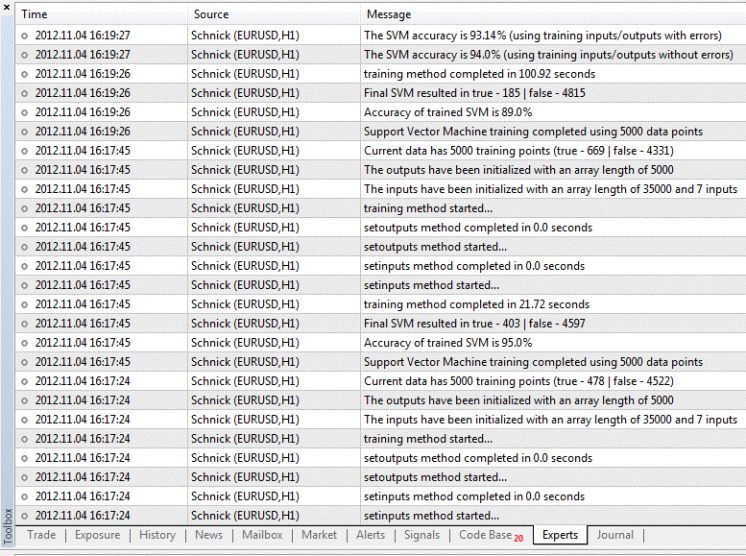Figure 5. The resulting expert log following the running of the "Schnick" script in the MetaTrader 5.

### Demo Versions

A full version of the above code can be downloaded from Code Base, however this script can only be run in your terminal if you have purchased a full version of the Support Vector Machine Learning tool from the Market. If you only have a demo version of this tool downloaded, you will be limited to using the tool via the strategy tester. To allow testing of the "Schnick" code using the demo version of the tool, I have rewritten a copy of the script into an Expert Advisor that can be deployed using the strategy tester. Both of these code versions can be downloaded by following the links below:

• Full Version - Using a Script that is deployed in the MetaTrader 5 terminal (requires a purchased version of the Support Vector Machine Learning Tool)

• Demo Version - Using an Expert Advisor that is deployed in the MetaTrader 5 strategy tester (requires only a demo version of the Support Vector Machine Learning Tool)

### How Can Support Vector Machines be used in the Market?

Admittedly, the Schnick example discussed above is quite simple, however there are quite a few similarities that can be drawn between this example and using the support vector machines for technical market analysis.

Technical analysis is fundamentally about using historical market data to predict future price movements. In the same way within the schnick example, we were using the observations made by past scientists to predict whether a new animal is a schnick or not. Further, the market is plagued with noise, errors and statistical outliers that make the use of a support vector machine an interesting concept.

The basis for a significant number of technical analysis trading approaches involve the following steps:

1. Monitoring several indicators
2. Identifying what conditions for each indicator correlates with a potentially successful trade
3. Watch each of the indicators and assess when they all (or most) are signalling a trade

It is possible to adopt a similar approach to use support vector machines to signal new trades in a similar way. The support vector machine learning tool was developed with this in mind. A full description of how to use this tool can be found in the Market, so I will only give a quick overview. The process for using this tool is as follows: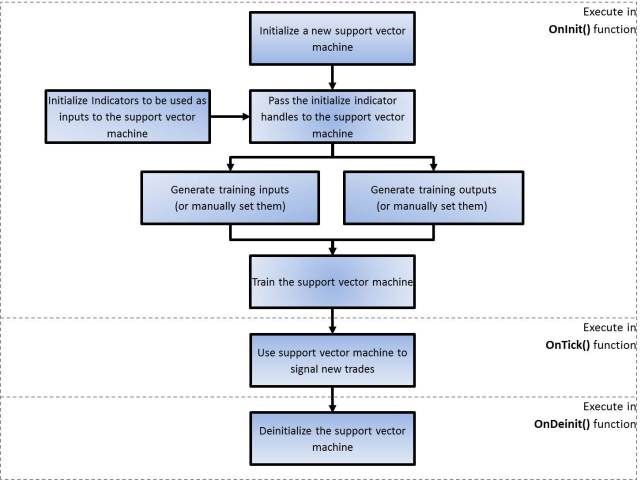Figure 6. The block diagram showing the process for implementing the support vector machine tool in an Expert Advisor

Before you can use the Support Vector Machine Learning Tool, it is important to first understand how the training inputs and outputs are generated.

### How are Training Inputs Generated?

So, the indicators you want to use as inputs have been already been initialized as well as your new support vector machine. The next step is to pass the indicator handles to your new support vector machine and instruct it on how to generate the training data. This is done by calling the setIndicatorHandles() function. This function allows you to pass the handles of initialized indicators into the support vector machine. This is done by passing and integer array containing the handles. The two other inputs for this function is the offset value and the number of data points.

The offset value denotes the offset between the current bar and the starting bar to be used in generating the training inputs and the number of training points (denoted by N) sets the size your training data. The diagram below illustrates how to use these values. An offset value of 4 and an N value of 6 will tell the support vector machine to only use the bars captured in the white square to generate training inputs and outputs. Similarly, an offset value of 8 and an N value of 8 will tell the support vector machine to only use the bars captured in the blue square to generate training inputs and outputs.

Once the setIndicatorHandles() function has been called, it is possible to call the genInputs() function. This function will use the indicator handles to passed to generate an array of input data to be used for training.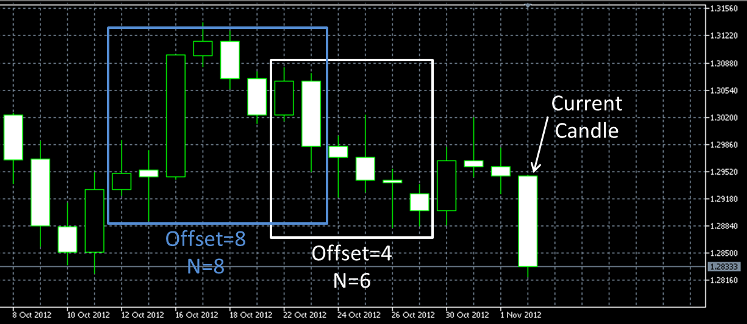Figure 7. Candle chart illustrating the values of Offset and N

### How are Training Outputs Generated?

Training outputs are generated by simulating hypothetical trades based on historical price data and determining whether such a trade would have been successful or unsuccessful. In order to do this, there are a few parameters that are used to instruct the support vector machine learning tool how to assess a hypothetical trade as either successful or unsuccessful.

The first variable is OP_TRADE. The value of this can either be BUY or SELL and will correspond to either hypothetical buy or sell trades. If the value of this is BUY, then when generating the outputs it will only look at the potential success of hypothetical buy trades. Alternatively, if the value of this is SELL, then when generating the outputs it will only look at the potential success of hypothetical sell trades.

The next values used is the Stop Loss and Take Profit for these hypothetical trades. The values are set in pips and will set the stop and limit levels for each of the hypothetical trades.

The final parameter is the trade duration. This variable is measured in hours and will ensure that only trades that are complete within this maximum duration will be deemed successful. The reason for including this variable is to avoid the support vector machine signalling trades in a slow moving sideways market.

### Considerations to Make When Choosing Inputs

It is important to put some thought into the input selection when implementing support vector machines in your trading. Similar the Schnick example, it is important to choose an input that would be expected to have similar across difference incidences. For example, you may be tempted to use a moving average as an input, however since the long term average price tends to change quite dramatically over time, a moving average in isolation may not be the best input to use. This is because there won't be any significant similarity between the moving average value today and the moving average values six months ago.

Assume we are trading EURUSD and using a support vector machine with a moving average input to signal 'buy' trades. Say the current price is 1.10, however it is generating training data from six months ago when the price was 0.55. When training the support vector machine, the pattern it finds may only lead to a trade being signaled when the price is around 0.55, since this is the only data it knows. Therefore, your support vector machine may never signal a trade until the price drops back down to 0.55.

Instead, a better input to use for the support vector machine may be a MACD or a similar oscillator because the value of the MACD is independent of the average price level and only signals relative movement. I recommend you experiment with this to see what produces the best results for you.

Another consideration to make when choosing inputs is ensuring that the support vector machine has an adequate snapshot of an indicator to signal a new trade. You may find in your own trading experience that a MACD is only useful when you have the past five bars to look at, as this will show a trend. A single bar of the MACD may be useless in isolation unless you can tell if it is heading up or down. Therefore, it may be necessary to pass the past few bars of the MACD indicator to the support vector machine.There are two possible ways you can do this:

1. You can create a new custom indicator that uses the past five bars of the MACD indicator to calculate a trend as a single value. This custom indicator can then be passed to the support vector machine as a single input, or

2. You can use the previous five bars of the MACD indicator in the support vector machine as five separate inputs. The way to do this is to initialize five different instances of the MACD indicator. Each of the indicators can be initialized with a different offset from the current bar. Then the five handles from the separate indicators can be passed to the support vector machine. It should be noted, that option 2 will tend to cause longer execution times for your Expert Advisor. The more inputs you have, the longer it will take to successfully train.

### Implementing Support Vector Machines in and Expert Advisor

I have prepared an Expert Advisor that is an example of how someone could potentially use support vector machines in their own trading (a copy of this can be downloaded by following this link https://www.mql5.com/en/code/1229). Hopefully the Expert Advisor will allow you to experiment a little with support vector machines. I recommend you copy/change/modify the Expert Advisor to suit your own trading style. The EA works as follows:

1. Two new support vector machines are created using the svMachineTool library. One is set up to signal new 'Buy' trades and the other is set up to signal new 'Sell' trades.

2. Seven standard indicators are initialized with each of their handles stored to an integer array (Note: any combination of indicators can be used as inputs, they just need to be passed to the SVM in a single integer array).

3. The array of indicator handles is passed to the new support vector machines.

4. Using the array of indicator handles and other parameters, historical price data is used to generate accurate inputs and outputs to be used for training the support vector machines.

5. Once all of the inputs and outputs have been generated, both of the support vector machines are trained.

6. The trained support vector machines are used in the EA to signal new 'buy' and 'sell' trades. When a new 'buy' or 'sell' trade is signaled, the trade opens along with manual Stop Loss and Take Profit orders.

The initialization and training of the support vector machine are executed within the onInit() function. For your reference, this segment of the svTrader EA has been included below with notes.

```#property copyright "Copyright 2011, MetaQuotes Software Corp."
#property version   "1.00"

#property indicator_buffers 7

//+---------Support Vector Machine Learning Tool Functions-----------+
//| The following #import statement imports all of the support vector
//| machine learning tool functions into the EA for use. Please note, if
//| you do not import the functions here, the compiler will not let you
//| use any of the functions
//+------------------------------------------------------------------+
#import "svMachineTool.ex5"
enum ENUM_OPTION {OP_MEMORY,OP_MAXCYCLES,OP_TOLERANCE};
int  initSVMachine(void);
void setIndicatorHandles(int handle,int &indicatorHandles[],int offset,int N);
void setParameter(int handle,ENUM_OPTION option,double value);
bool genInputs(int handle);
bool setInputs(int handle,double &Inputs[],int nInputs);
bool setOutputs(int handle,bool &Outputs[]);
bool training(int handle);
bool classify(int handle);
bool classify(int handle,int offset);
bool classify(int handle,double &iput[]);
void  deinitSVMachine(void);
#import

//+-----------------------Input Variables----------------------------+
input int            takeProfit=100;      // TakeProfit level measured in pips
input int            stopLoss=150;        // StopLoss level measured in pips
input double         hours=6;             // The maximum hypothetical trade duration for calculating training outputs.
input double         risk_exp=5;          // Maximum simultaneous order exposure to the market
input double         Tolerance_Value=0.1; // Error Tolerance value for training the SVM (default is 10%)
input int            N_DataPoints=100;    // The number of training points to generate and use.

//+---------------------Indicator Variables--------------------------+
//| Only the default indicator variables have been used here. I
//| recommend you play with these values to see if you get any
//| better performance with your EA.
//+------------------------------------------------------------------+
int bears_period=13;
int bulls_period=13;
int ATR_period=13;
int mom_period=13;
int MACD_fast_period=12;
int MACD_slow_period=26;
int MACD_signal_period=9;
int Stoch_Kperiod=5;
int Stoch_Dperiod=3;
int Stoch_slowing=3;
int Force_period=13;

int         tickets[];
bool        Opn_B,Opn_S;
datetime    New_Time;
int         handleB,handleS;
double      Vol=1;
//+------------------------------------------------------------------+
//|                                                                  |
//+------------------------------------------------------------------+
int OnInit()
{
New_Time=0;
int handles[];ArrayResize(handles,7);
//+------------------------------------------------------------------+
//| The following statements are used to initialize the indicators to be used for the support
//| vector machine. The handles returned are stored to an int[] array. I have used standard
//| indicators in this case however, you can also you custom indicators if desired
//+------------------------------------------------------------------+
handles=iBearsPower(Symbol(),0,bears_period);
handles=iBullsPower(Symbol(),0,bulls_period);
handles=iATR(Symbol(),0,ATR_period);
handles=iMomentum(Symbol(),0,mom_period,PRICE_TYPICAL);
handles=iMACD(Symbol(),0,MACD_fast_period,MACD_slow_period,MACD_signal_period,PRICE_TYPICAL);
handles=iStochastic(Symbol(),0,Stoch_Kperiod,Stoch_Dperiod,Stoch_slowing,MODE_SMA,STO_LOWHIGH);
handles=iForce(Symbol(),0,Force_period,MODE_SMA,VOLUME_TICK);

//----------Initialize, Setup and Training of the Buy-Signal support vector machine----------
handleB=initSVMachine();                             // Initializes a new SVM and stores the handle to 'handleB'
setIndicatorHandles(handleB,handles,0,N_DataPoints); // Passes the initialized indicators to the SVM with the desired offset
// and number of data points
setParameter(handleB,OP_TOLERANCE,Tolerance_Value);  // Sets the maximum error tolerance for SVM training
genInputs(handleB);                                  // Generates inputs using the initialized indicators
genOutputs(handleB,BUY,stopLoss,takeProfit,hours);   // Generates the outputs based on the desired parameters for taking hypothetical trades

//----------Initialize, Setup and Training of the Sell-Signal support vector machine----------
handleS=initSVMachine();                             // Initializes a new SVM and stores the handle to 'handleS'
setIndicatorHandles(handleS,handles,0,N_DataPoints); // Passes the initialized indicators to the SVM with the desired offset
// and number of data points
setParameter(handleS,OP_TOLERANCE,Tolerance_Value);  // Sets the maximum error tolerance for SVM training
genInputs(handleS);                                  // Generates inputs using the initialized indicators
genOutputs(handleS,SELL,stopLoss,takeProfit,hours);  // Generates the outputs based on the desired parameters for taking hypothetical trades
//----------
training(handleB);   // Executes training on the Buy-Signal support vector machine
training(handleS);   // Executes training on the Sell-Signal support vector machine
return(0);
}```

Additional capability was built into the support vector machine learning tool for the more advanced users out there. The tool allows users to pass in their own custom input data and output data (as in the Schnick example). This allows you to custom design your own criteria for support vector machine inputs and outputs, and manually pass in this data to train it. This opens up the opportunity to use support vector machines in any aspect of your trading.

It is not only possible to use support vector machines to signal new trades, but it can also be used to signal the closing of trades, money management, new advanced indicators etc. However to ensure you don’t receive errors, it is important to understand how these inputs and outputs are to be structured.

Inputs: Inputs are passed to SVM as a 1 dimensional array of double values. Please note that any input you create must be passed in as a double value. Boolean, integer, etc. must all be converted into a double value before being passed into the support vector machine. The inputs are required in the following form. For example, assume we are passing in inputs with 3 inputs x 5 training points. To achieve this, our double array must be 15 units long in the format:

| A1 | B1 | C1 | A2 | B2 | C2 | A3 | B3 | C3 | A4 | B4 | C4 | A5 | B5 | C5 |

It is also necessary to pass in a value for the number of inputs. In the case, N_Inputs=3.

Outputs: outputs are passed in as an array of Boolean values. These boolean values are the desired output of the SVM corresponded to each of the sets of inputs passed in. Following the above example, say we have 5 training points. In this scenario, we will pass in a Boolean array of output values that is 5 units long.

General Notes:

• When generating your own inputs and outputs, be sure that the length of your arrays matches the values you pass in. If they don’t match, an error will be generated notifying you of the discrepancy. For example, if we have passed in N_Inputs=3, and inputs is an array of length 16, an error will be thrown (since, a N_inputs value of 3 will mean that the length of any input array will need to be a multiple of 3). Similarly, ensure that the number of sets of inputs and the number of outputs that you pass in are equal. Again, if you have N_Inputs=3, length of inputs of 15 and a length of outputs of 6, another error will be thrown (as you have 5 sets of inputs and 6 outputs).

• Try to ensure you have enough variation in your training outputs. For example, if you pass in 100 training points, which means an output array of length 100, and all of the values are false with only one true, then the differentiation between the true case and the false case is not sufficient enough. This will tend to lead to the SVM training very fast, but the final solution being very poor. A more diverse training set will often lead to a more affective SVM.

Attached files |
schnick.mq5 (10.8 KB)
schnick_demo.mq5 (11.39 KB)
Last comments | Go to discussion (6)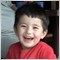| 19 Dec 2012 at 19:47
MetaQuotes:

New article Machine Learning: How Support Vector Machines can be used in Trading is published:

Thank you very much for you article.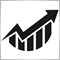| 24 Mar 2013 at 08:52
Great article, thanks!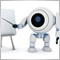| 29 May 2013 at 17:29

Very useful for implementing SVM in trading!

Great work!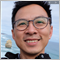| 28 Nov 2013 at 07:36
great stuff!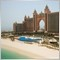| 16 Sep 2015 at 06:55
great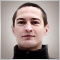Interview with Evgeny Gnidko (ATC 2012)

The Expert Advisor of Evgeny Gnidko (FIFO) currently seems to be the most stable one at the Automated Trading Championship 2012. This trading robot entered TOP-10 at the third week remaining one of the leading Expert Advisors ever since.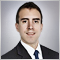Interview with Juan Pablo Alonso Escobar (ATC 2012)

"Everyone who is struggling with programming and who were not able to participate in this year's competition, know that it becomes a lot easier in time", said Juan Pablo Alonso Escobar (JPAlonso), the hero of today's interview.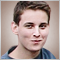Interview with Mariusz Zarnowski (ATC 2012)

As December 28 is approaching, the list of leaders of the Automated Trading Championship 2012 is becoming clearer. With only two weeks to go until the end of the Championship, Mariusz Zarnowski (zrn) from Poland stands a good chance to be in the top three. His EA has already demonstrated how it can triple the initial deposit in just a couple of weeks.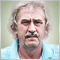Interview with Alexandr Artapov (ATC 2012)

It was during the second week of the Championship when the Expert Advisor of Alexandr Artapov (artall) found itself on the third position trading EURUSD and EURJPY. Then it briefly left TOP-10 to appear again after one month of struggle for survival. As it turned out, this trading robot is still having something up its sleeve.